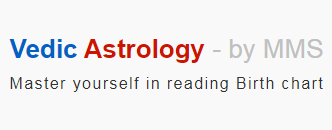# Ascendant

Ascendant : We know that Ascendant in a horoscope indicates the time of birth of a person. The Zodiacal sign that the Sun was rising in the Eastern horizon at the time of birth of a person is known as Ascendant sign of the person.

On any given day, all the planets will be almost in the same sign. But, only Ascendant will keep changing the signs for every two hours, approx. As all the houses are referred from Ascendant, natural benefic may become functional malefic or Badhakathipathi and natural malefic may become functional benefic depending on the the placement of Ascendant. Similarly, Yoga (Luck) may become Dosha (Blemish) and Dosha may become Yoga based on the Ascendant Sign. Such is the importance of Ascendant sign.

Now, let us discuss in detail how Ascendant is determined based on Month and Time of birth, and Sunrise time at the place of birth.

We know that Sun travels through one Sign (Rasi) in every Tamil month. The First day of the Year or New Year is celebrated when Sun enters Aries in Chittirai Month. So, obviously Chittirai will be the first month of 12 months.

Thus, the Sun will be in Aries in the month of Chittirai, will be in Taurus in Vaikasi, in Gemini in Aani and finally in Pisces in Panguni which is the last month.

The picture below shows in which Sign the Sun will be in every month. As the English months span across two months, it is better to determine the Ascendant sign using Tamil months.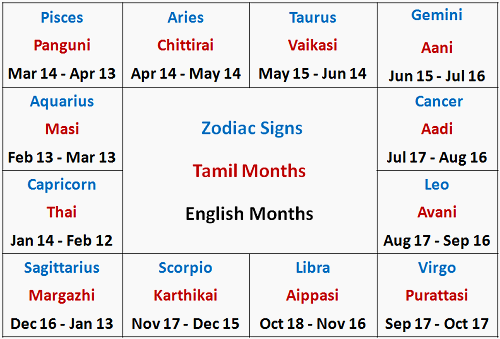We know that Earth takes 24 hours or 1440 minutes to rotate 360 degrees on its axis. So, it will take 1440 / 360 = 4 minutes to rotate one degree. Since each sign is 30 degrees, Earth will take 30 x 4 = 120 minutes i.e. 2 hours to rotate one sign.

When the Sun rises where we are on Earth, we will be in the same sign as the Sun. As the Earth rotates, the Sun will rise in successive signs every 2 hours.

Let's look at the above two together. Let us take Avani month (Aug 17th - Sep 16th). The Sun will be in Leo during this month. When the sun rises where we are, we will also be in Leo. As the Earth rotates on its axis, the Sun will rise (ascending) in Virgo 2 hours later, in Libra 4 hours later and so on. During the last two hours, i.e. 22 to 24 hours, the sun will rise in Cancer. The next day morning the Sun will again rise in Leo.

In Vedic astrology, a "day" refers to the time elapsed between two successive sunrise times. For instance, if the sunrise is at 5.57 am today, then 5.57 am today to 5.57 am tomorrow is considered as a day.The Sunrise time can be obtained from Phanchang (Ephemeris) or e-Panchange or daily calender. Now, let us find out how to calculate the Ascendant sign using all these information.

Let us say a person is born at 7.00 am in Avani month. And let the Sunrise time be 5.57 am on that day. As the month is Avani, Sun will be in Leo for the first 2 hours i.e., from 5.57 am to 7.56 am. As the person is born at 7.00 am, the Ascendant should be marked in Leo sign.

But, if the person is born at 10.00 am in Avani, then Ascendant will be in Libra. It is because the Sun will be in Leo between 5.57 am to 7.56 am, in Virgo between 7.57 am to 9.56 am and in Libra between 9.57 am to 11.56 am. So, the Ascendant should be marked in Libra as the Sun will be in Libra at 10.00 am.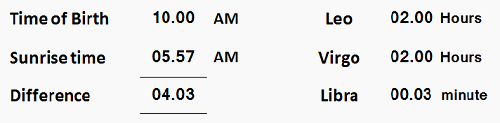This is a rough method of determining the Ascendant Sign because the Date of Birth is not accounted for. Also, Earth takes less than 2 hours to rotate some signs and more than 2 hours to rotate some signs. Now, let us consider all these factors and find out how to determine the Ascendant sign accurately.

As the Earth transits every day, it will be at 1st degree of the Sign on Day 1 of the month, at 15th degree on day 15 and at 30th degree on dat 30. So, on the last day of the month, the time required by the Earth to rotate the Sign will be 1 degree x 4 min = 4 minutes only.

For instance, on 1st of Chittirai, Earth will take 30 x 4 = 120 minutes i.e., 2 hrs to rotate the Aries sign. If it is 15th morning, then it will be 15 x 4 = 60 min. And, if it is 30th morning, then Earth will take 1 x 4 = 4 min only to rotate the Aries sign.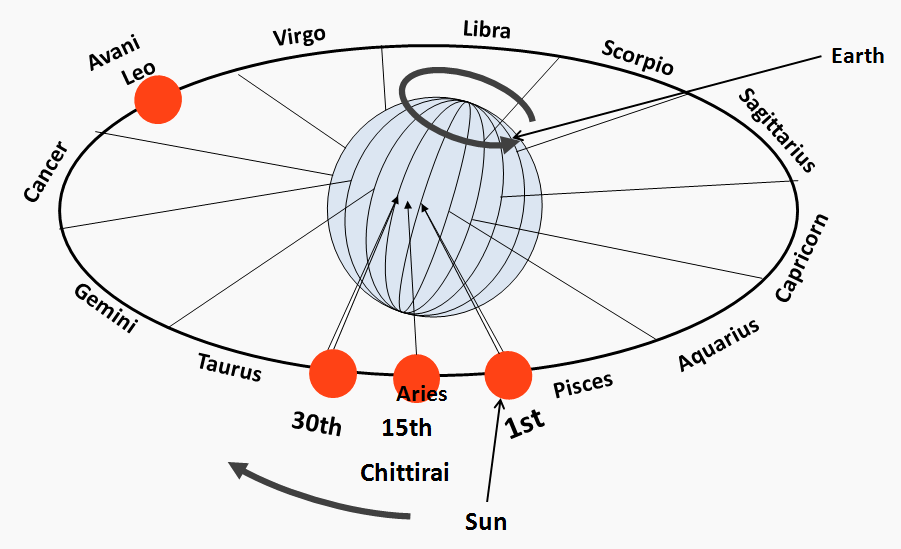As the Earth is tilted at 23.5 degree on its axis, the time taken by the Earth to rotate each sign will differ. The time taken by the Earth to rotate each Sign is called "Rasimanam" in Tamil, meaning "Measurement of Sign".

The Rasimanam or the Rotation-Time is given below, both in Nazhigai and Minutes. The Sum total of minutes will be 1440 which is nothing but 24 hours. Similarly, the Sum total of Nazhigai will be 60 which makes one day.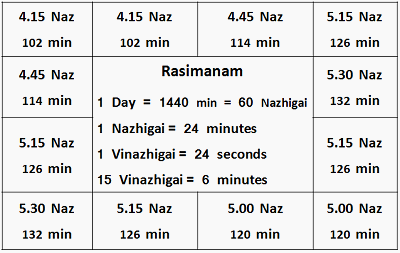Now, let us determine the Ascendant based on the Date of birth, Time and Place, Sunrise time and Rasimanam or Cycle time. For this,

1. First, find out the Elapsed Time (ET) between Sunrise Time (SrT) and Time of Birth (TOB) in minutes.
2. Next, calculate the time required by the Earth to rotate the First Sign. Time required to rotate the other signs should be taken from the table
3. Finally, subtract the Rotation-time of each Sign from ET until it cannot be subtracted. The Sign whose Rotation-Time cannot be subtracted will be the Ascendant Sign
Ex 1 - Let us consider the DOB and TOB of a person is Aug 17th or Avani 1st, 10.00 AM. And let the Sunrise time be 5.57 AM on that day.
1. ET = TOB - SrT = 10.00 - 05.57 = 4.03 Hrs = 4 x 60 + 3 = 243 min
2. As the person is born on 1st Avani, the balance days in Avani is 31. Earth Rotation-time for the First sign Leo will be = 31/31 x 126 = 126 min
3. 243 - 126 (First sign Leo) = 117 min. The Rotation-time for the next Sign Virgo is 120 min. As 120 cannot be subtracted from 117, the Ascendant Sign will be Virgo

Ex 2 - Let us consider the Date and Time of birth of a person is Sep 1st or Avani 16th, 10.00 AM. And let the Sunrise time be 6.01 AM on that day. Sun will be in Leo as the month is Avani. No of Days in Avani is 31.

1. ET = TOB - SrT = 10.00 - 6.01 = 3.59 Hrs = 3 x 60 + 59 = 239 min
2. As the Date of birth is 16th morning, the balance days in Avani is 31 - 15 = 16. Earth Rotation-time for the First sign Leo will be = 16/31 x 126 = 65.03 = 65 min
3. Subtracting the Rotation-time for the First sign Leo and the next Sign Virgo, the balance time = 239 - (65.03+120) = 54 min. The Rotation time for the next Sign Libra time is 120 min. As 120 cannot be subtracted from 54, the Ascendant Sign will be Libra

Ex 3 - Let us consider the Date and Time of birth of a person is Sep 16th or Avani 31st, 6.05 PM. And let the Sunrise time be 6.05 AM on that day.

1. ET = TOB - SrT = 18.05 - 6.05 = 12.00 Hrs = 12 x 60 = 720 min
2. As the Date of birth is 31st evening, the balance days in Avani is just 0.5 days. Earth Traverse-time for the First sign Leo will be = 0.5/31 x 126 = 2.03 = 2 min
3. Balance time = 720 - 2 (Leo) - 120 (Virgo) - 120 (Libra) - 126 (Scorpio) - 132 (Sagittarius) - 126 (Capricorn) = 94 min. The next Sign Aquarius time is 114 min. As 114 cannot be subtracted from 94, the Ascendant Sign will be Aquarius
Now that we know how to determine the Ascendant sign, it is always better to ensure it is marked correctly in the horoscope because the entire forecast depends on the Ascendant.

As a start, you can verify whether the Ascendant is correctly marked in your horoscope according to your Date, Time and Place of birth and Sunrise time at your place of birth.

Note : In any country where day and night are somewhat equal in length, the above method will hold good. In countries such as the United States and Russia, daylight hours are longer in summer and shorter in winter. Besides, in countries like Norway it is 6 months day and 6 months night. Therefore, for those born in such countries, Ascendant should be predicted using appropriate Rasimanam.